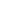Original research

# Smart edge detection technique in X-ray images for improving PSNR using sobel edge detection algorithm with gaussian filter in comparison with laplacian algorithm

## Abstract

Aim:The aim of this study is to propose smart edge detection techniques in x-ray images for improving PSNR using the sobel edge detection algorithm and comparing it with the laplacian algorithm. Materials and methods: For the design of edge detection technique to improve PSNR Sobel edge detection algorithm is used along with the gaussian filter and it is compared with the laplacian algorithm. Sobel edge detection algorithm and laplacian algorithm are the two groups considered in this study. For each group, the sample size is 20 and the total sample size is 40. Sample size calculation was done using clinicalc.com by keeping g-power at 80%, confidence interval at 95%, and the threshold at 0.05%. Result: When comparing the two algorithms, it is clear that the Sobel edge detection algorithm has a higher mean PSNR value of 39.15db than the laplacian algorithm 36.79db. It is observed that the Sobel edge detection algorithm performed better than the laplacian algorithm by performing an independent sample t-test.The p value is 0.09 which is greater than the normal value(p>0.05). Conclusion: Sobel edge detection has insignificantly greater PSNR when compared to the Laplacian algorithm.

## Imprint

C.Nithish karthick, P.Nirmala. Smart Edge Detection Technique in X-ray Images for Improving PSNR using Sobel Edge Detection Algorithm with Gaussian Filter in Comparison with Laplacian Algorithm. Cardiometry; Issue 25; December 2022; p.1751-1757; DOI: 10.18137/cardiometry.2022.25.17511757; Available from: https://www.cardiometry.net/issues/no25-december-2022/sobel-edge-detection-algorithm

## Keywords

Edge detection,   Sobel Edge Detection,   Laplacian Algorithm,   PSNR,   Image processing,   Innovative edge detection techniqueCurrent issueCardiometry's libraryFounders of Cardiometry